#Python股市数据分析教程——学会它，或可以实现半“智能”炒股 (Part 1)

12
111
21. 云栖社区>
2. 博客>
3. 正文

## Python股市数据分析教程——学会它，或可以实现半“智能”炒股 (Part 1)

【方向】 2016-12-20 14:32:39 浏览24290

## 获取并可视化股票数据

### 使用pandas从雅虎财经中获取数据

import pandas as pd
import pandas.io.data as web
import datetime

start = datetime.datetime(2016,1,1)
end = datetime.date.today()

apple = web.DataReader("AAPL", "yahoo", start, end)

type(apple)
C:\Anaconda3\lib\site-packages\pandas\io\data.py:35: FutureWarning:
The pandas.io.data module is moved to a separate package (pandas-datareader) and will be removed from pandas in a future version.
After installing the pandas-datareader package (https://github.com/pydata/pandas-datareader), you can change the import from pandas.io import data, wb to from pandas_datareader import data, wb.
FutureWarning)

pandas.core.frame.DataFrame
apple.head()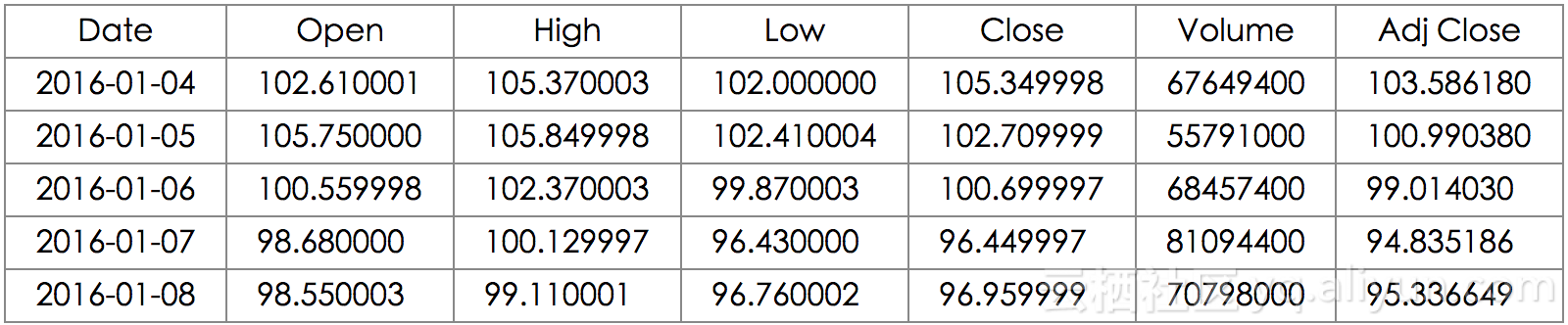### 股票数据可视化

import matplotlib.pyplot as plt

%matplotlib inline

%pylab inline
pylab.rcParams['figure.figsize'] = (15, 9)

apple["Adj Close"].plot(grid = True) 
Populating the interactive namespace from numpy and matplotlib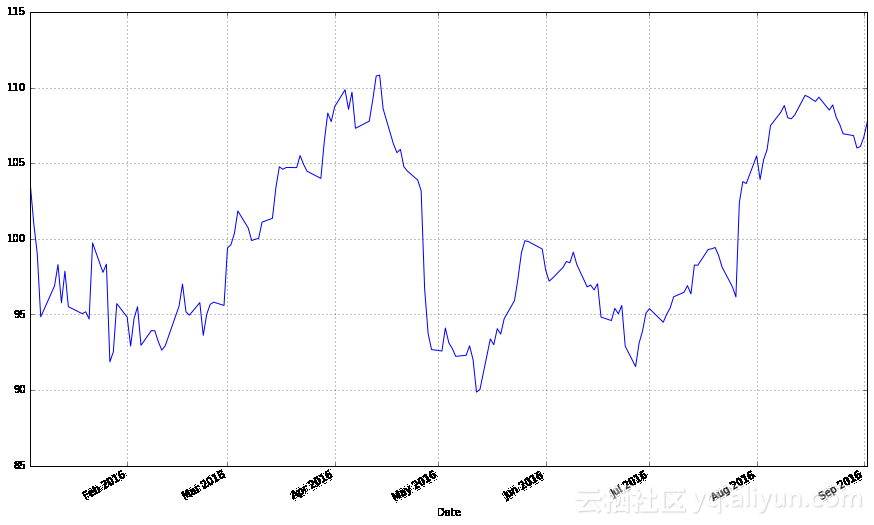from matplotlib.dates import DateFormatter, WeekdayLocator,\
DayLocator, MONDAY
from matplotlib.finance import candlestick_ohlc

def pandas_candlestick_ohlc(dat, stick = "day", otherseries = None):
mondays = WeekdayLocator(MONDAY)
alldays = DayLocator()
dayFormatter = DateFormatter('%d')

transdat = dat.loc[:,["Open", "High", "Low", "Close"]]
if (type(stick) == str):
if stick == "day":
plotdat = transdat
stick = 1
elif stick in ["week", "month", "year"]:
if stick == "week":
transdat["week"] = pd.to_datetime(transdat.index).map(lambda x: x.isocalendar())
elif stick == "month":
transdat["month"] = pd.to_datetime(transdat.index).map(lambda x: x.month)
transdat["year"] = pd.to_datetime(transdat.index).map(lambda x: x.isocalendar())
grouped = transdat.groupby(list(set(["year",stick])))
plotdat = pd.DataFrame({"Open": [], "High": [], "Low": [], "Close": []})

for name, group in grouped:
plotdat = plotdat.append(pd.DataFrame({"Open": group.iloc[0,0],
"High": max(group.High),
"Low": min(group.Low),
"Close": group.iloc[-1,3]},
index = [group.index]))
if stick == "week": stick = 5
elif stick == "month": stick = 30
elif stick == "year": stick = 365

elif (type(stick) == int and stick >= 1):
transdat["stick"] = [np.floor(i / stick) for i in range(len(transdat.index))]
grouped = transdat.groupby("stick")
plotdat = pd.DataFrame({"Open": [], "High": [], "Low": [], "Close": []})
for name, group in grouped:
plotdat = plotdat.append(pd.DataFrame({"Open": group.iloc[0,0],
"High": max(group.High),
"Low": min(group.Low),
"Close": group.iloc[-1,3]},
index = [group.index]))

else:
raise ValueError('Valid inputs to argument "stick" include the strings "day", "week", "month", "year", or a positive integer')

fig, ax = plt.subplots()
if plotdat.index[-1] - plotdat.index < pd.Timedelta('730 days'):
weekFormatter = DateFormatter('%b %d')
ax.xaxis.set_major_locator(mondays)
ax.xaxis.set_minor_locator(alldays)
else:
weekFormatter = DateFormatter('%b %d, %Y')
ax.xaxis.set_major_formatter(weekFormatter)

ax.grid(True)

candlestick_ohlc(ax, list(zip(list(date2num(plotdat.index.tolist())), plotdat["Open"].tolist(), plotdat["High"].tolist(),
plotdat["Low"].tolist(), plotdat["Close"].tolist())),
colorup = "black", colordown = "red", width = stick * .4)

if otherseries != None:
if type(otherseries) != list:
otherseries = [otherseries]
dat.loc[:,otherseries].plot(ax = ax, lw = 1.3, grid = True)

ax.xaxis_date()
ax.autoscale_view()
plt.setp(plt.gca().get_xticklabels(), rotation=45, horizontalalignment='right')

plt.show()

pandas_candlestick_ohlc(apple)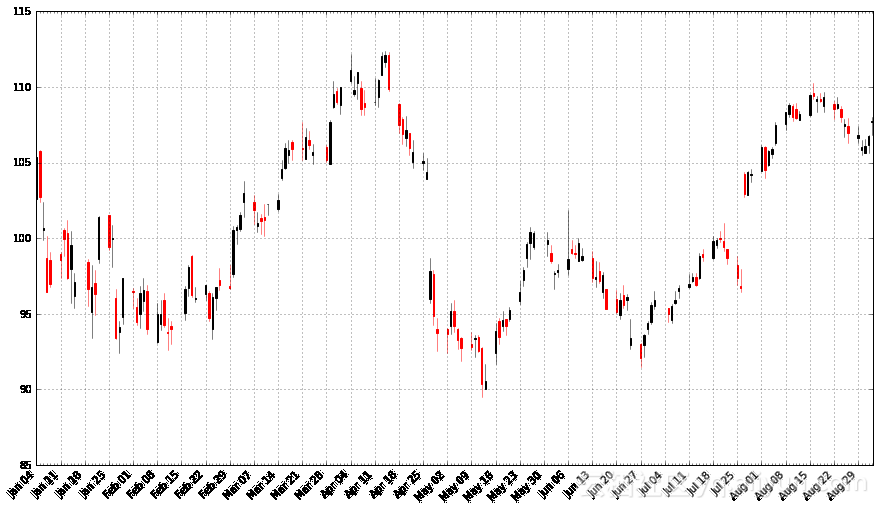microsoft = web.DataReader("MSFT", "yahoo", start, end)

stocks.head()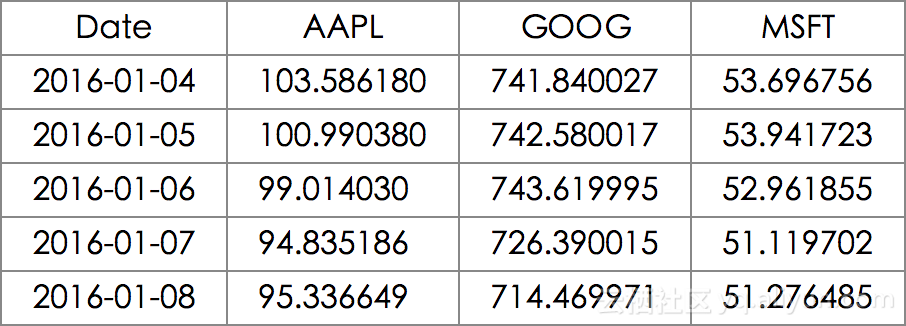stocks.plot(grid = True)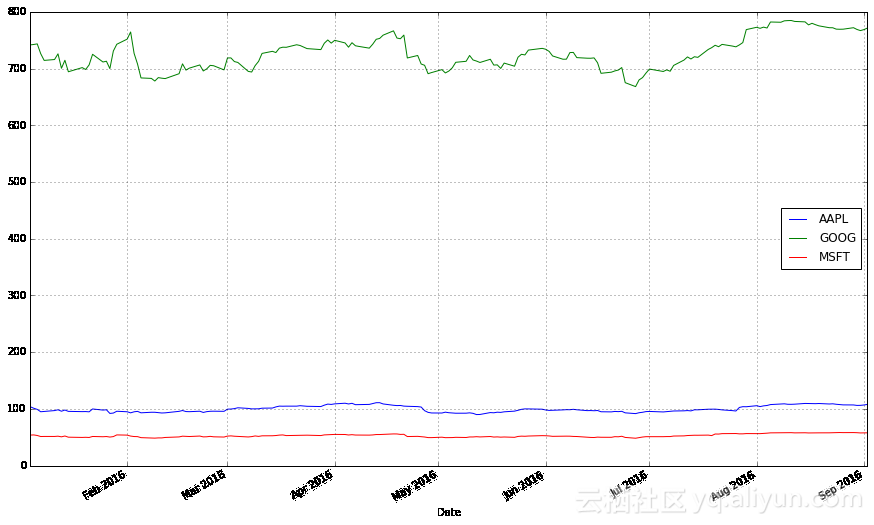stocks.plot(secondary_y = ["AAPL", "MSFT"], grid = True)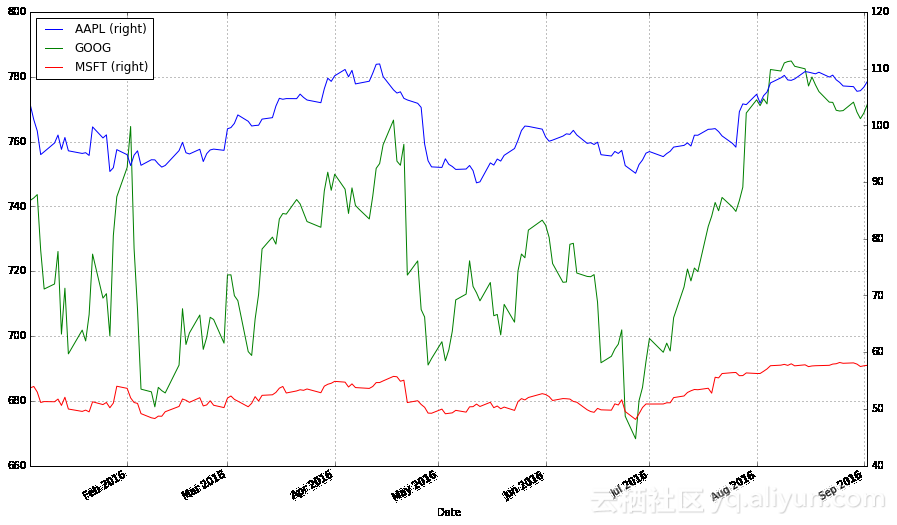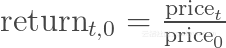stock_return = stocks.apply(lambda x: x / x)
stock_return.head()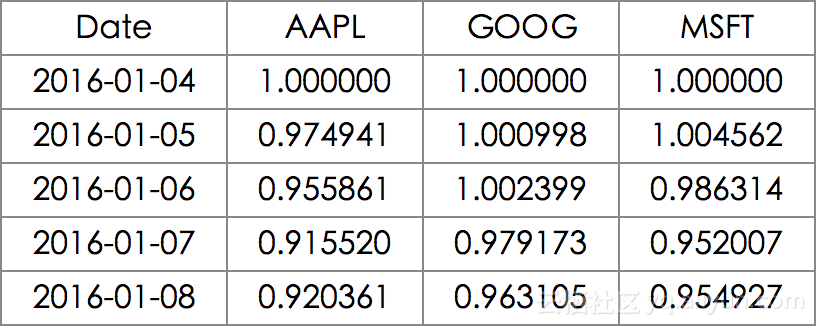stock_return.plot(grid = True).axhline(y = 1, color = "black", lw = 2)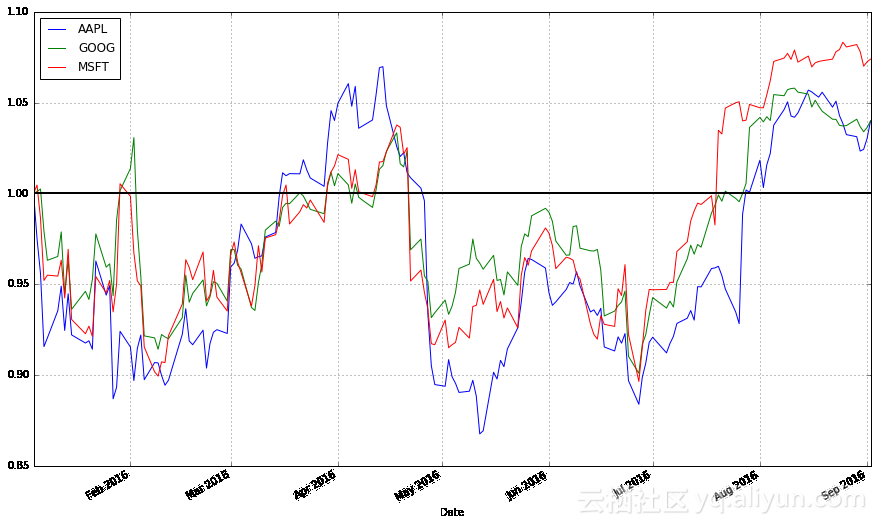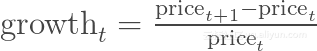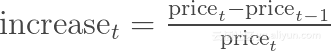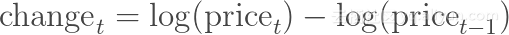（这里的log为自然对数，我们的定义并不关心使用的是第t天与第t-1天的对数差值还是第t+1天与第t天的对数差值。）使用对数差值的好处在于，这种差值可以理解为股价的百分比变化，且不依赖于计算过程中分数的分母。

import numpy as np

stock_change = stocks.apply(lambda x: np.log(x) - np.log(x.shift(1)))
stock_change.head()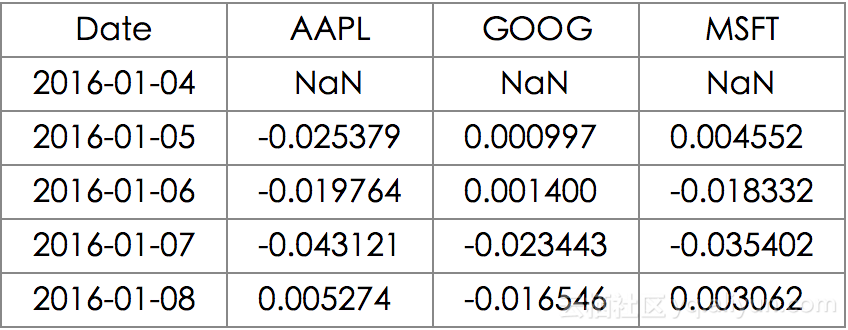stock_change.plot(grid = True).axhline(y = 0, color = "black", lw = 2)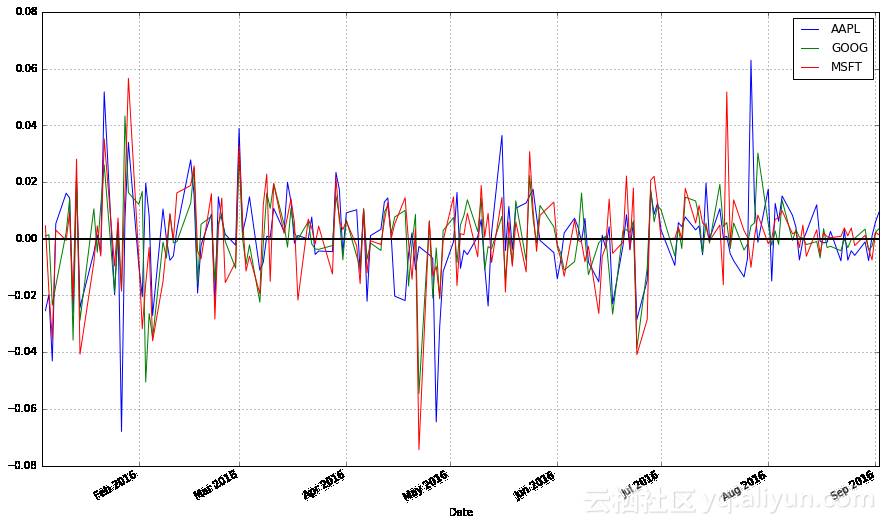## 移动均线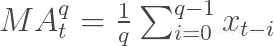pandas提供了轻松计算移动均线的功能。下面的代码展示了这部分功能，我首先为苹果股票创建了一条20天（1个月）均线，随后，将其与股票数据一同绘制在图表中。

apple["20d"] = np.round(apple["Close"].rolling(window = 20, center = False).mean(), 2)
pandas_candlestick_ohlc(apple.loc['2016-01-04':'2016-08-07',:], otherseries = "20d")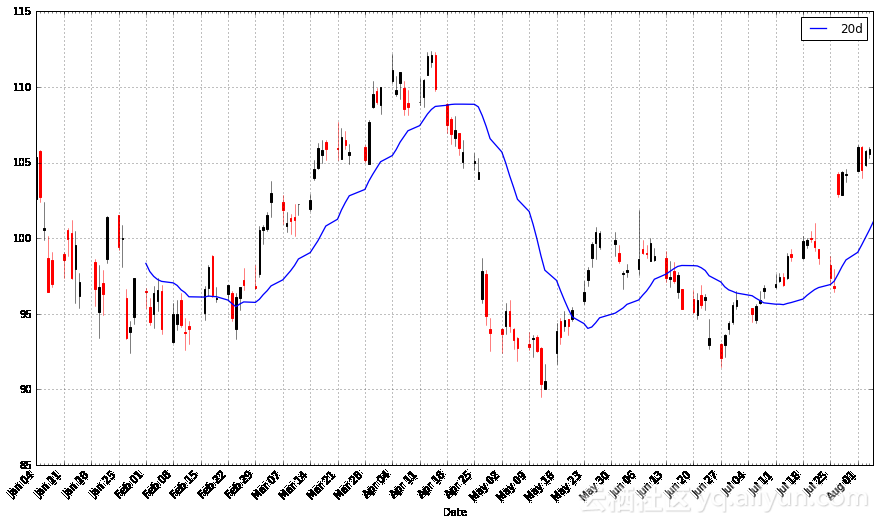start = datetime.datetime(2010,1,1)
apple = web.DataReader("AAPL", "yahoo", start, end)
apple["20d"] = np.round(apple["Close"].rolling(window = 20, center = False).mean(), 2)

pandas_candlestick_ohlc(apple.loc['2016-01-04':'2016-08-07',:], otherseries = "20d")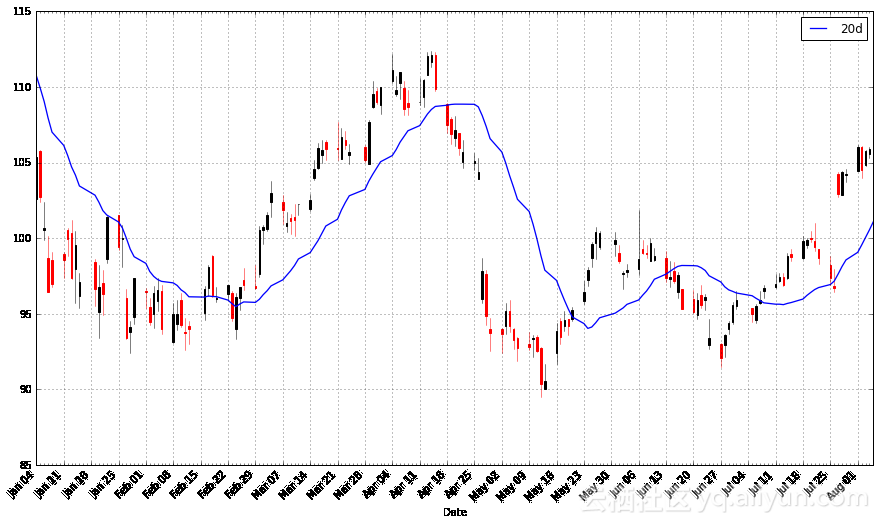apple["50d"] = np.round(apple["Close"].rolling(window = 50, center = False).mean(), 2)
apple["200d"] = np.round(apple["Close"].rolling(window = 200, center = False).mean(), 2)

pandas_candlestick_ohlc(apple.loc['2016-01-04':'2016-08-07',:], otherseries = ["20d", "50d", "200d"])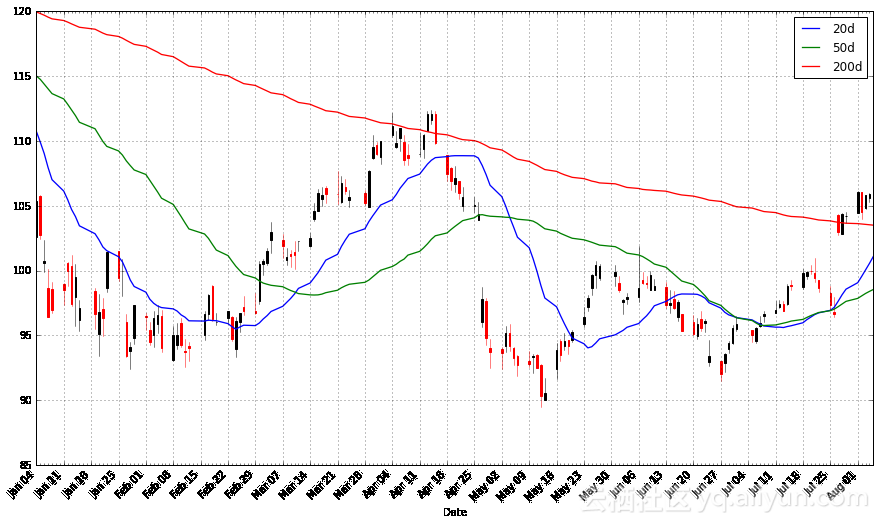【方向】
+ 关注